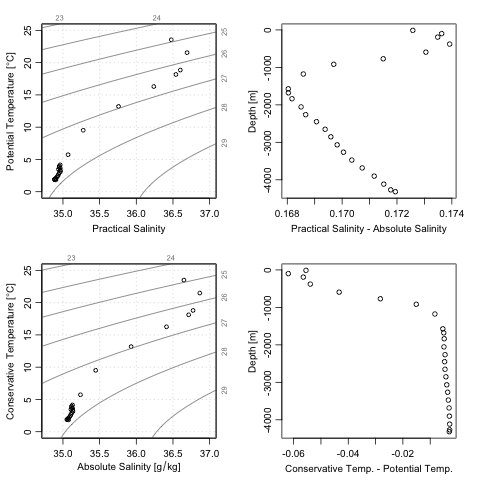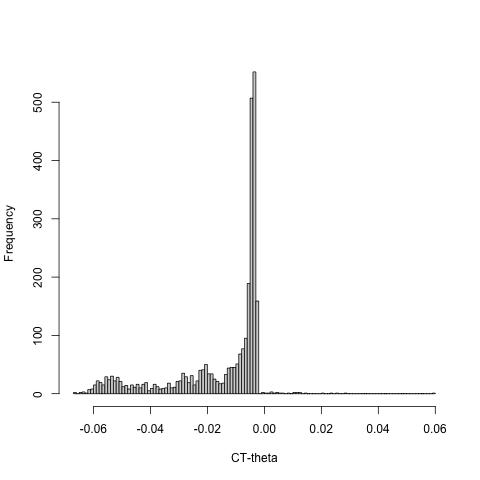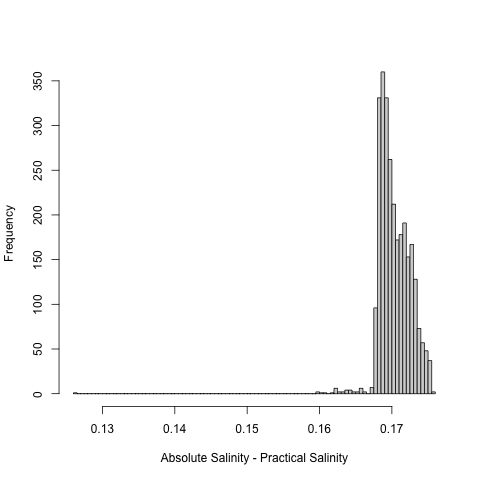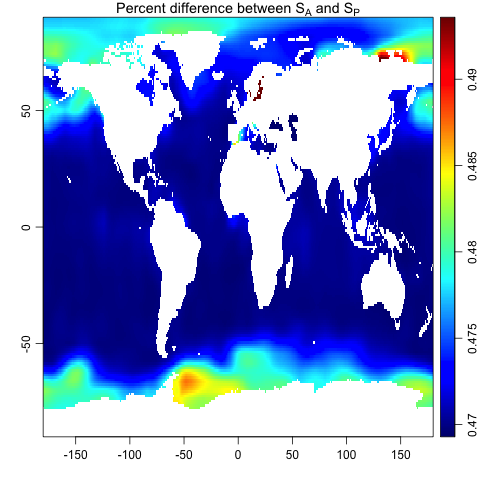# gsw

#### 2022-10-16

Abstract. The gsw package provides an R implementation of the Gibbs SeaWater toolbox for the calculation of seawater properties, based on the GSW-C framework1. This vignette outlines how to use gsw alone or as part of the oce package (Kelley, Richards, and Layton 2021).

# 1 Introduction

In recent years, thermodynamic considerations have led to improved formulae for the calculation of seawater properties (IOC, SCOR, and IAPSO 2010; Millero 2010; Pawlowicz et al. 2012), an important component of which is the Gibbs-SeaWater (GSW) toolbox (McDougall and Barker 2020). The gsw package is an R version of GSW, which may be used independently or within the more general oce package (Kelley, Richards, and Layton 2021).

This vignette sketches how to use gsw. Readers are assumed to be familiar with oceanographic processing, and at least somewhat familiar with GSW. A good resource for learning more about GSW is http://www.teos-10.org, which provides technical manuals for the Matlab version of GSW http://www.teos-10.org/pubs/gsw/html/gsw_contents.html, along with white papers and links to the growing peer-reviewed literature on the topic.

The gsw framework uses function wrappers that connect R with the C version of the Gibbs Seawater library. This yields high processing speed. By minimizing transliteration errors, it also increases reliability. In a further effort to increase reliability, GSW-R makes tests against the check values provided on the webpages that document GSW-Matlab.

By design, the documentation of gsw functions is spare, amounting mainly to an explanation of function arguments and return values, with most other details being provided through hyperlinks to the GSW reference documentation. The idea is to avoid duplication and to encourage users to consult the technical materials linked to the GSW functions mimicked in gsw. The GSW system is somewhat complex, and analysts owe it to themselves to learn how it works, and also to develop an appreciation for its scientific context by consulting various documents at http://www.teos-10.org, including expansive white papers and pointers to the growing peer-reviewed literature (Wright et al. 2011; McDougall and Barker 2020; Graham and McDougall 2012).

# 2 Using gsw independent of oce

Suppose a water sample taken at pressure (For practical reasons, gsw goes beyond SI to incorporate oceanographic units, such as decibars for pressure.) 100 dbar, longitude 188E and latitude 4N, reveals Practical Salinity 35 and in-situ temperature 10$$^\circ$$C (ITS-90). Then the Absolute Salinity may be calculated as follows.

library(gsw)
SA <- gsw_SA_from_SP(SP=35, p=100, longitude=188, latitude=4)

This yields SA=35.1655491 [g/kg], which can then be used to calculate Conservative Temperature as follows.

CT <- gsw_CT_from_t(SA=SA, t=10, p=100)

The above yields CT=9.9782488 [$$^\circ$$C]. Readers familiar with GSW will recognize the function and argument names, and are likely to find the other functions needed for their work among the roughly sixty functions that gsw provides.

# 3 Using gsw within oce

Many oce plotting functions have an argument named eos that can be set to the string "unesco" to get the older seawater formulation, or to "gsw" to get the newer one. For example, the section dataset provided by oce holds a sequence of CTD casts in the North Atlantic. Individual casts may be selected by index, so a TS diagram of the station at index 100 (south of Cape Cod in 4000 m of water) can be plotted as follows.

library(oce)
data(section)
ctd <- section[["station", 100]]
Slim <- c(34.8, 37.0)
Tlim <- c(0, 25)
par(mfcol=c(2,2))
plotTS(ctd, Slim=Slim, Tlim=Tlim, eos="unesco")
plotTS(ctd, Slim=Slim, Tlim=Tlim, eos="gsw")
plot(ctd[["SA"]] - ctd[["salinity"]], ctd[["z"]],
xlab="Practical Salinity - Absolute Salinity", ylab="Depth [m]")
plot(ctd[["CT"]] - ctd[["theta"]], ctd[["z"]],
xlab="Conservative Temp. - Potential Temp.", ylab="Depth [m]")Comparison of UNESCO and GSW equations of state, for bottle sampling at 36.3N and 69.4W in 1993. Top left: TS diagram with UNESCO equation of state, showing Potential Temperature versus Practical Salinity. Bottom left: TS diagram with GSW equation of state, showing Conservative Temperature versus Absolute Salinity. Top right: Absolute Salinity minus Practical Salinity, as a function of depth. Bottom right: Conservative Temperature minus Potential Temperature, as a function of depth.

Most hydrography-related functions of oce provide this eos argument for selecting the seawater formulation. This includes functions for plotting and for calculating. In addition, most of the objects within oce have accessors that can return temperature and salinity in either the UNESCO or GSW scheme. For example, the ratio of Conservative Temperature to UNESCO-formulated potential temperature $$\theta$$ for all the CTD profiles in section is constructed with

f <- section[["CT"]] - section[["theta"]]
hist(f, main="", breaks=100, xlab="CT-theta")Histogram of difference between Conservative Temperature and Potential temperature for the 124 stations of a cross-Atlantic cruise at approximately 36N.

A salinity comparison is constructed with

f <- section[["SA"]] - section[["salinity"]]
hist(f, main="", breaks=100, xlab="Absolute Salinity - Practical Salinity")Histogram of difference between Absolute Salinity and Practical Salinity for the 124 stations of a cross-Atlantic cruise at approximately 36N.

An examination of worldwide spatial patterns is also informative, with the following producing such a graph.

library(oce)
data("levitus", package="ocedata")
SSS <- levitus$SSS dim <- dim(SSS) ll <- expand.grid(lon=levitus$longitude, lat=levitus$latitude) SA <- gsw_SA_from_SP(levitus$SSS, 0, ll$lon, ll$lat)
per <- 100 * (1 - levitus$SSS / SA) imagep(levitus$longitude, levitus\$latitude, per, col=oceColorsJet,
zlim=quantile(per, c(0.001, 0.999), na.rm=TRUE))
title(expression("Percent difference between " * S[A] * " and " * S[P]))

Note the use of quantile-specified scales for the images, the colour mappings of which would otherwise be controlled by isolated low-saline waters, yielding little to see in the wider expanses of the world ocean; for a broader context, see e.g. McDougall and Barker (2020).Map of the relative difference between Absolute Salinity and Practical Salinity at the sea surface.

1. This version of GSW-R is based on GSW-C as it existed on 2021-07-06 in github commit 9c10670e89fce906da2cebce3399d73c054e769e; see https://github.com/TEOS-10/GSW-C.↩︎